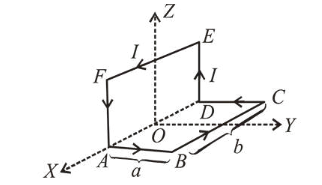# A wire carrying current I is bent in the shape A B C D E F A as shown,

Question:

A wire carrying current $I$ is bent in the shape $A B C D E F A$ as shown, where rectangle $A B C D A$ and $A D E F A$ are perpendicular to each other. If the sides of the rectangles are of lengths $a$ and $b$, then the magnitude and direction of magnetic moment of the loop $A B C D E F A$ is :1. (1) $a b I$, along $\left(\frac{\hat{j}}{\sqrt{2}}+\frac{\hat{k}}{\sqrt{2}}\right)$

2. (2) $\sqrt{2} a b I$, along $\left(\frac{\hat{j}}{\sqrt{2}}+\frac{\hat{k}}{\sqrt{2}}\right)$

3. (3) $\sqrt{2} a b I$, along $\left(\frac{\hat{j}}{\sqrt{5}}+\frac{2 \hat{k}}{\sqrt{5}}\right)$

4. (4) $a b I$, along $\left(\frac{\hat{j}}{\sqrt{5}}+\frac{2 \hat{k}}{\sqrt{5}}\right)$

Correct Option: 1

Solution:

(1) Magnetic moment of loop $A B C D$,

$M_{1}=$ area of loop $\times$ current

$\vec{M}_{1}=(a b I)(\hat{j})$                  (Here, $a b=$ area of rectangle)

Magnetic moment of loop $D E F A$,

$\vec{M}_{2}=(a b I)(\hat{i})$c

Net magnetic moment,

$\vec{M}=\vec{M}_{1}+\vec{M}_{2} \Rightarrow \vec{M}=a b I(\hat{i}+\hat{j})$

$\Rightarrow|\vec{M}|=\sqrt{2} a b I\left(\frac{\hat{j}}{\sqrt{2}}+\frac{\hat{k}}{\sqrt{2}}\right)$